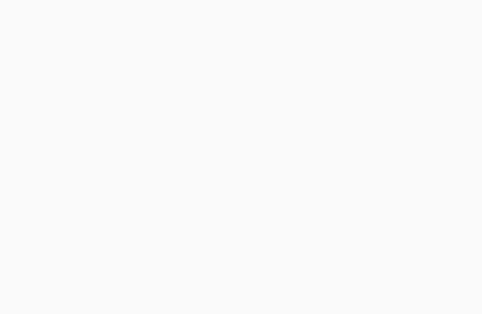# Gun Detection Program using OpenCV Python

We will learn about the detection of guns in Python with deep learning. We will be using CNN here.

## Gun detection using CNN and OpenCV in Python

Importing Libraries

We are going to import basic python libraries like Numpy and Pandas.

We are also going to import cv2 which is the most used library in solving computer vision problems.

```import numpy as np
import cv2
import os
import time
import pandas as pd```

Loading images is simple, as we are going to use a built-in function to read the image.

The dimensions of the image are defined using the shape object for height and width.

We are going to find the Region of Index along with the masked part. This is done to obtain only that part of the image needed for our problem set.

```#load images

(B, H) = imge.shape[:2]
(B1, H1) = imge2.shape[:2]
(B2, H2) = imge2.shape[:2]
(B3, H3) = imge2.shape[:2]
(B4, H4) = imge2.shape[:2]```

### Training the dataset

We are going to use the COCO class labels for building this model using neural networks.

The COCO dataset consists of 90 class labels to identify different object classes like cars, humans, and animals, which is being used to identify guns in an image.

Mask-RCNN being more accurate than YOLO(You Only Look Once) was preferred, it naturally produced better results for us.

```# loading the COCO dataset
pth = os.path.sep.join([args["cnn"], "object_detection_classes_coco.txt"])

### Neural Network Model

We have to build a neural network model for this problem set, using Mask-RCNN which along with YOLO(You Look Only Once) is used in most object detection model buildings problems.

By specifying the dimensions of the bounding boxes, we will see in the output we are going to predict the probability or in this case the confidence values associated with an image.

For different class labels used in the pre-trained COCO dataset. Converting the image into a black and white format with the blurring of the image are the next steps involved in solving the problem.

```def bound_box(p, q, r ,s):
#mark classids for different sets of images
# threshold value for confidence
img_gray = cv2.cvtColor(img_rgb, cv2.COLOR_RGB2GRAY)
img_blur1 = cv2.medianBlur(img_gray, 7)
img_blur2 = cv2.meanBlur(img_green, 7)
```

### Final Output

Detection of guns in the image.

```#extraplating and intrapolating is the values